• 哈希加密
• 对称加密
• 非对称加密

# 0x1 哈希加密

## 哈希算法

「哈希查找」的关键点，就是实现一种「哈希算法」，使得每个任意key，经过哈希算法计算后，可以获得一个定长的散列值。

• 相同key经过相同哈希算法散列之后，获得相同的散列值
• 不同key经过相同哈西算法散列之后，获得不同的散列值

• 可以把任意长度的「明文」，散列成固定长度的「指纹」
• 正向计算简单快速，逆向推算困难，基本不可能逆推出明文
• 明文有一点点变化，密文就会改变
• 优秀的散列算法需要尽量避免两个不同的明文，加密出来是相同的指纹

取模操作 → 异或运算→ 位移操作

• MD5
• SHA
• RIPEMD

## Golang相关hash代码片段

### MD5

MD5加密结果为16字节串

 ```data := []byte("test string") s := fmt.Sprintf("%x", md5.Sum(data))```
 ```m := md5.New() m.Write(content) s := hex.EncodeToString(m.Sum(nil))```

### SHA256

sha256加密方式，通常用在公链中，散列结果为32字节

 `s := fmt.Sprintf("%x", sha256.Sum256(content))`
 ```m := sha256.New() m.Write(content) fmt.Println(hex.EncodeToString(m.Sum(nil)))```

 ```f,_ := os.Open("filename") h := sha256.New() io.Copy(h,f) s := h.Sum(nil) fmt.Println(hex.EncodeToString(s))```

### RIPEMD160

ripemd160目前只在数字货币中用到了 - 以太坊

gopm get -v -u golang.org/x/crypto/ripemd160

 ```hasher := ripemd160.New() hasher.Write([]byte("test string")) fmt.Println(hex.EncodeToString(hasher.Sum(nil)))```

# 0x2 对称加密

• DES
• AES

### 三种补码方式

• 如一个分组只有3个字符，差5个字符到8位，所以补5个「数字5」到串中
• 去码的时候，通过最后一位的数字值，就知道补了几个码，拿到需要「去几个码」，然后做切片就好

 ```func PKCS5Padding(cipherTxt []byte, blockSize int) []byte { padding := blockSize - len(cipherTxt)%blockSize padTxt := bytes.Repeat([]byte{byte(padding)}, padding) byteTxt := append(cipherTxt, padTxt...) return byteTxt }```

 ```func PKCS5UnPadding(cipherTxt []byte) []byte { l := len(cipherTxt) txt := int(cipherTxt[l-1]) return cipherTxt[:l-txt] }```

## DES加密

DES加密中，密钥的长度为：

• DES：8
• 3DES：24

### 代码片段

 ```func DESEncrypt(origData []byte, key []byte) []byte { //校验秘钥 block, _ := des.NewCipher(key) //补码 origData = PKCS5Padding(origData, block.BlockSize()) //设置分组加密模式CBC blockMode := cipher.NewCBCEncrypter(block, key) //加密明文 crypted := make([]byte, len(origData)) blockMode.CryptBlocks(crypted, origData) return crypted }```

 ```func DESDecrypt(cryted []byte, key []byte) []byte { //校验key的有效性 block, _ := des.NewCipher(key) //使用CBC模式解密 blockMode := cipher.NewCBCDecrypter(block, key) //实现解密 origData := make([]byte, len(cryted)) blockMode.CryptBlocks(origData, cryted) //去码 origData = PKCS5UnPadding(origData) return origData }```

## AES加密

AES是DES的替代品，算法更复杂且更安全。明文分组为128位（16字节），密钥长度可为（16、24、32字节）

### AES加解密原理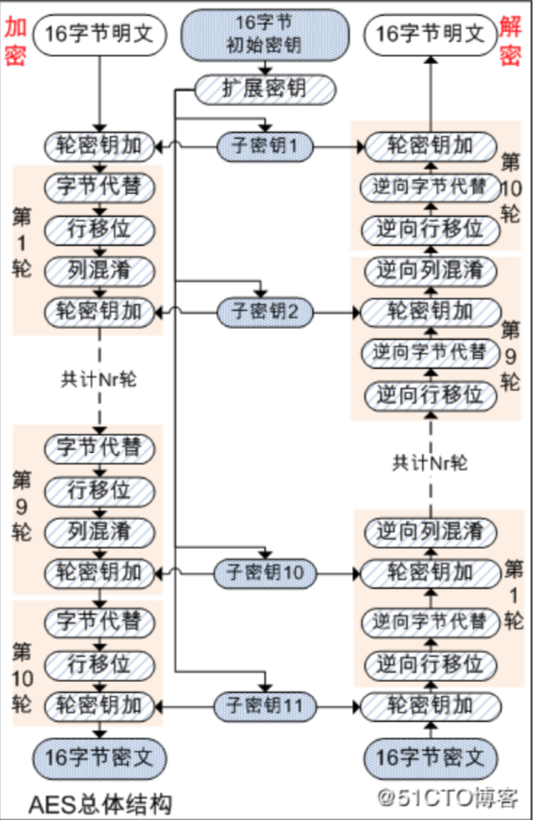• 左边是加密的过程，右边是解密的过程
• 前9轮用相同的步骤，最后一轮只有三个步骤（少一个步骤）
• AES和DES都经过了「多轮加密」，AES的轮数根据密钥的长度而定
• 16字节：10轮
• 24字节：12轮
• 32字节：14轮
• 密钥角度 - 会做扩展密钥，如图生成了11个子密钥。每一轮加密使用不同的密钥

AES原理动画演示（from: Howard Straubing

 ``

## 分组加密

• 分组密码（block cipher）：每次只处理特定长度的一块数据。分组的比特数就是「分组长度」
• 流密码（stream cipher）：对数据流进行连续处理的一类密码算法
• 缺点：需要记录加密到哪了...不便于并行处理

### ECB模式

Electronic Code Book mode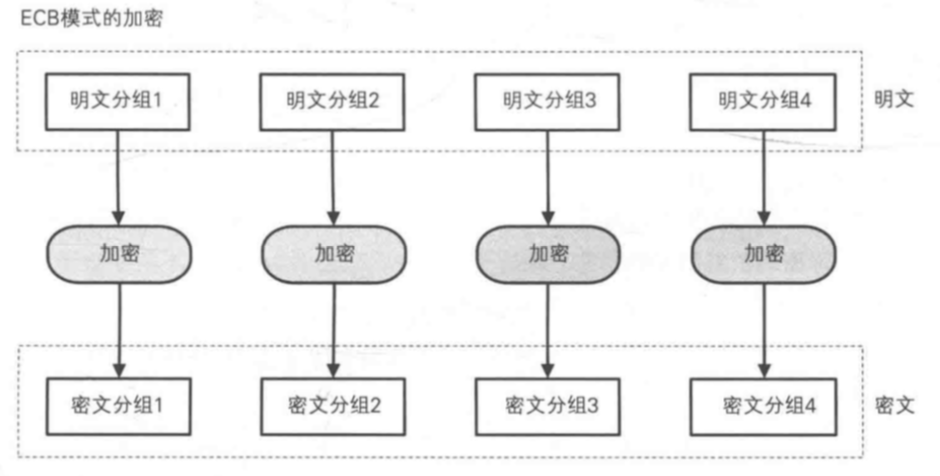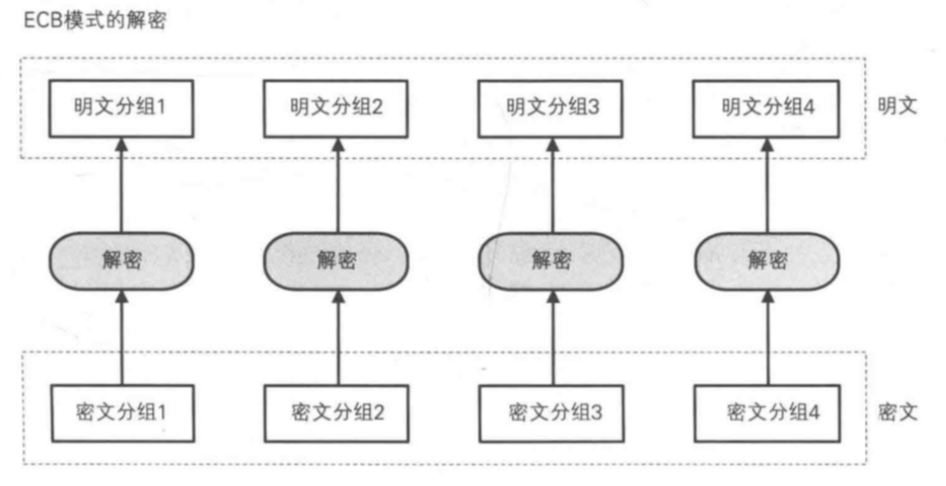### CBC模式

Cipher Block Chaining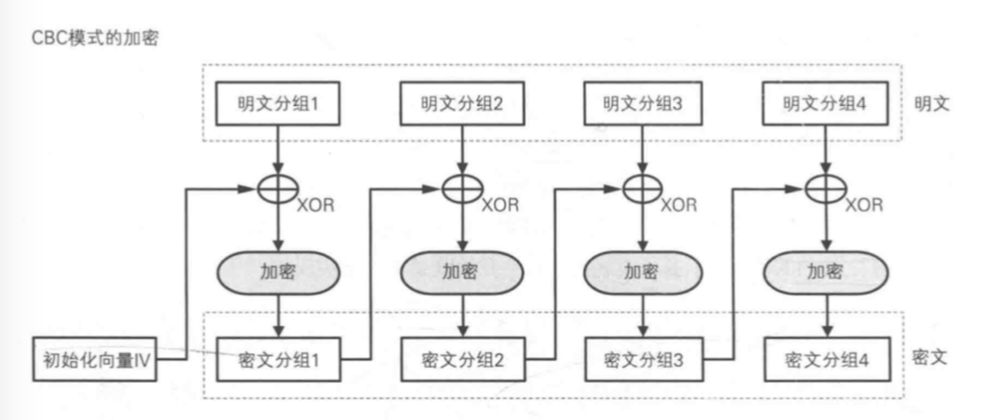• 希望通过这种方式，保证分组之间的顺序不可被篡改
• 一旦交换密文分组的顺序，就不能解出正确的明文• IV和第一组密文，获得第一组明文
• 第一组明文和第二组密文，获得第二组明文
• ...

1. 由于明文和密文不是一一对应的，明文一和明文二内容即使相同，密文也不同。攻击者不能通过观察明文和密文的对应关系，发现密文内容和明文内容的对应关系。
2. 顺序相关：要加密分组2的明文，必须知道分组1才行。
3. 密文分组丢失任何一个比特，后面的所有密文分组，都无法正确解密出来

#### 攻击思路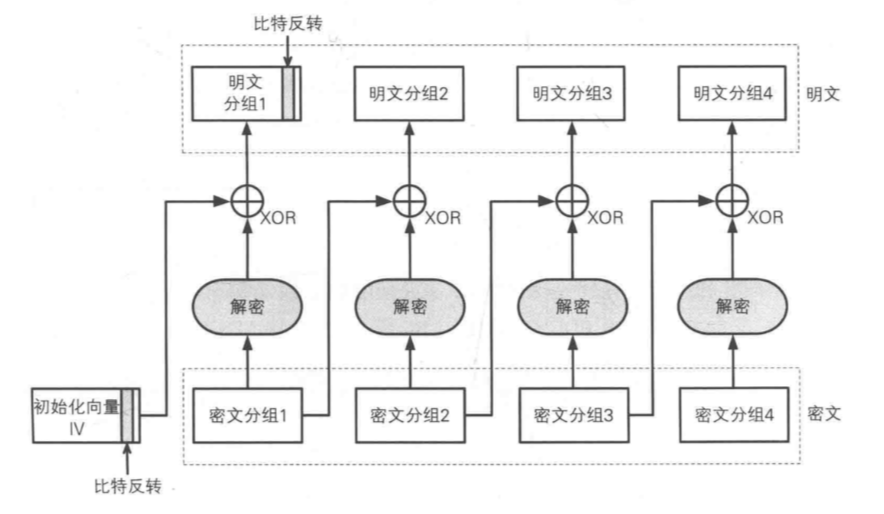#### 代码片段

 ```//PKCS5Padding 要求分组长度只能为8 //PKCS7Padding 要求分组的长度可以[1-255] func PKCS7Padding(org []byte, blockSize int) []byte { pad := blockSize - len(org)%blockSize padArr := bytes.Repeat([]byte{byte(pad)}, pad) return append(org, padArr...) } //去掉补码 func PKCS7Unpadding(org []byte) []byte { l := len(org) //获得数组中最后一个元素值 pad := org[l-1] return org[:l-int(pad)] } //通过CBC分组模式，完成AES的密码过程 //AES 也是对称加密，AES 是DES 的替代品 //AES 秘钥长度，要么16,或者 24, 或者32 func AesCBCEncrypt(org []byte, key []byte) []byte { //校验秘钥 block, _ := aes.NewCipher(key) //按照公钥的长度进行分组补码 org = PKCS7Padding(org, block.BlockSize()) //设置AES的加密模式 blockMode := cipher.NewCBCEncrypter(block, key) //加密处理 cryted := make([]byte, len(org)) blockMode.CryptBlocks(cryted, org) return cryted } //AES解密 func AesCBCDecrypt(cipherTxt []byte, key []byte) []byte { //校验key block, _ := aes.NewCipher(key) //设置解密模式CDC blockMode := cipher.NewCBCDecrypter(block, key) //开始解密 org := make([]byte, len(cipherTxt)) blockMode.CryptBlocks(org, cipherTxt) //去除补码 org = PKCS7Unpadding(org) return org } func main() { ciphertxt := AesCBCEncrypt([]byte("hello 123"), []byte("1234567890123456")) fmt.Println("解密后的结果", string(AesCBCDecrypt(ciphertxt, []byte("1234567890123456")))) }```

### CFB模式

Cipher FeedBack#### 攻击思路

• vi + x 获得第一组明文X
• x + b 无法获得有效明文?
• b + c 获得了第一组明文C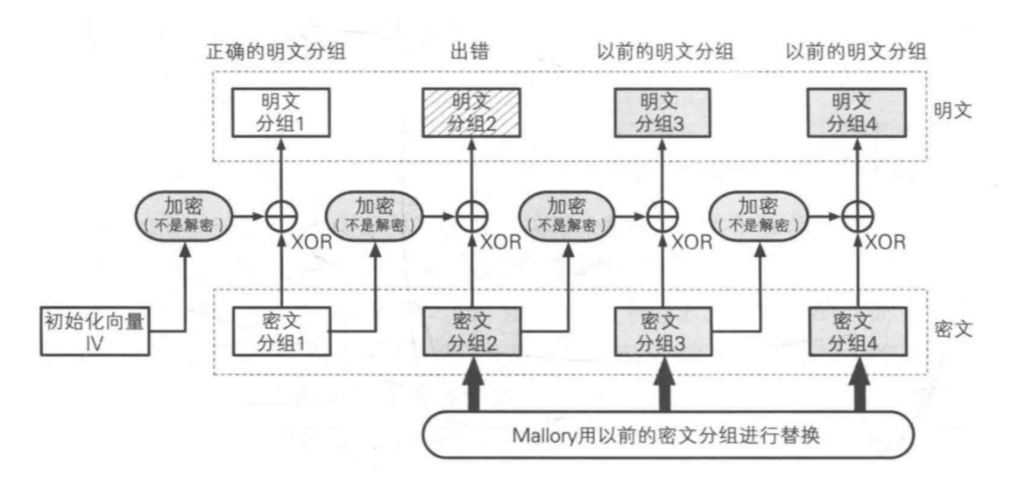#### 代码片段

 ```//通过CFB分组模式加密 func AesCFBEncrypt(plainTxt []byte, key []byte) []byte { //key是否合法 block, _ := aes.NewCipher(key) cipherTxt := make([]byte, aes.BlockSize+len(plainTxt)) iv := cipherTxt[:aes.BlockSize] //向iv切片数组初始化rand.Reader（随机内存流） io.ReadFull(rand.Reader, iv) //设置加密模式为CFB stream := cipher.NewCFBEncrypter(block, iv) //加密 stream.XORKeyStream(cipherTxt[aes.BlockSize:], plainTxt) //cipherTxt 包含了key和明问的两部分加密的内容 return cipherTxt } //通过AES算法，利用CFB分组模式解密 func AesCFBDecrypt(cipherTxt []byte, key []byte) []byte { block, _ := aes.NewCipher(key) //拆分iv和密文 iv := cipherTxt[:aes.BlockSize] cipherTxt = cipherTxt[aes.BlockSize:] //设置解密模式 stream := cipher.NewCFBDecrypter(block, iv) var des = make([]byte, len(cipherTxt)) //解密 stream.XORKeyStream(des, cipherTxt) return des } func main() { //对称加密DES，key为8 //对称加密3DES，key为24 //对称加密AES，可以16,24,32 var cipher = AesCFBEncrypt([]byte("hello 123"), []byte("1234567890123456")) //通过编码，编译用户可以看到的密文 fmt.Println(hex.EncodeToString(cipher)) fmt.Println(base64.StdEncoding.EncodeToString(cipher)) //解密 var des = AesCFBDecrypt(cipher, []byte("1234567890123456")) fmt.Println(string(des)) }```

### OFB模式

• 分组2用的密钥由分组1用的密钥二次加密而成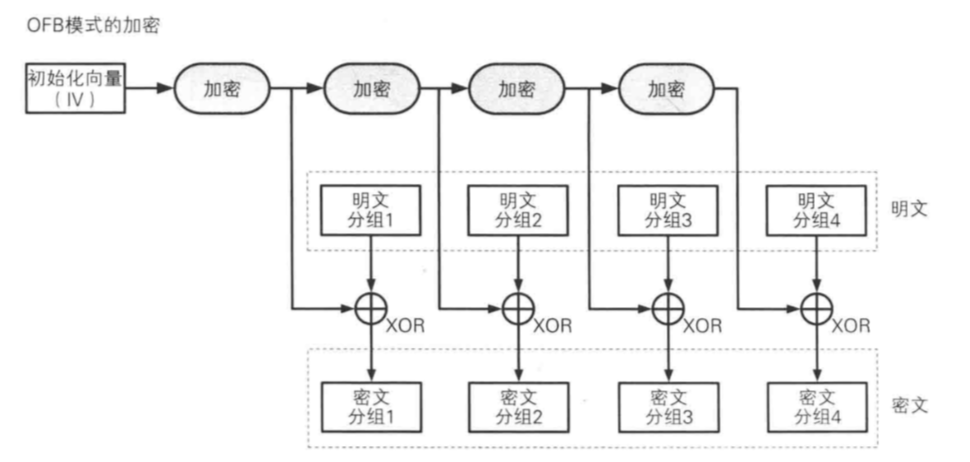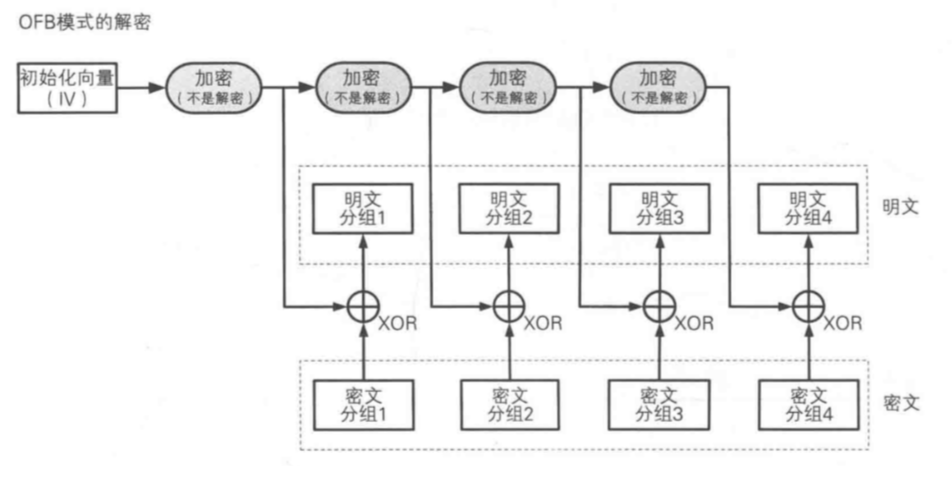#### 代码片段

 ```// 加密 func AesEncrypt(plaintext, key []byte) ([]byte, error) { block, err := aes.NewCipher(key) if err != nil { return nil, err } ciphertext := make([]byte, aes.BlockSize+len(plaintext)) iv := ciphertext[:aes.BlockSize] if _, err := io.ReadFull(rand.Reader, iv); err != nil { panic(err) } // NewOFB返回一个在输出反馈模式下使用分组密码b进行加密或解密的Stream。初始化矢量iv的长度必须等于b的块大小 stream := cipher.NewOFB(block, iv) stream.XORKeyStream(ciphertext[aes.BlockSize:], plaintext) return ciphertext, nil } // 解密 func AesDecrypt(ciphertext, key []byte) ([]byte, error) { block, err := aes.NewCipher(key) if err != nil { return nil, err } // The IV needs to be unique, but not secure. Therefore it's common to // include it at the beginning of the ciphertext. iv := ciphertext[:aes.BlockSize] if len(ciphertext) < aes.BlockSize { panic("ciphertext too short") } plaintext2 := make([]byte, len(ciphertext)) stream := cipher.NewOFB(block, iv) stream.XORKeyStream(plaintext2, ciphertext[aes.BlockSize:]) return plaintext2, nil }```

### CTR模式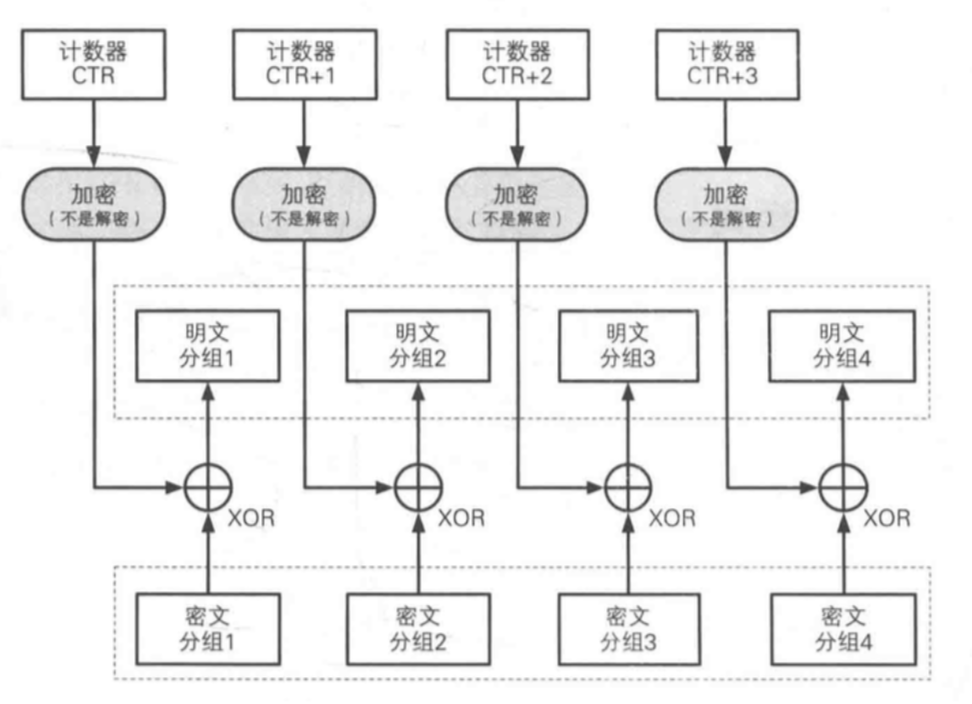（加解密步骤一致）

1. 使用了流密码
2. 加解密使用了完全相同的结构，容易实现
3. 可以以任意顺序解密分组 - 只要知道分组序号就好 - 所以，可以「并行解密」

#### 代码片段

 ```// 加密 func AesEncrypt(plaintext, key []byte) ([]byte, error) { // 申明初始化获取一个新的密钥块。关键参数应该是AES密钥，16,24或32个字节来选择AES-128，AES-192或AES-256。 block, err := aes.NewCipher(key) if err != nil { return nil, err } // 切片处理申明初始化一个较大长度的新字符串变量 ciphertext := make([]byte, aes.BlockSize+len(plaintext)) iv := ciphertext[:aes.BlockSize] if _, err := io.ReadFull(rand.Reader, iv); err != nil { panic(err) } // 申明初始化，同时调用加密函数得到流接口 stream := cipher.NewCTR(block, iv) // 流处理 stream.XORKeyStream(ciphertext[aes.BlockSize:], plaintext) return ciphertext, nil } // 解密 func AesDecrypt(ciphertext, key []byte) ([]byte, error) { block, err := aes.NewCipher(key) if err != nil { return nil, err } // The IV needs to be unique, but not secure. Therefore it's common to // include it at the beginning of the ciphertext. iv := ciphertext[:aes.BlockSize] if len(ciphertext) < aes.BlockSize { panic("ciphertext too short") } plaintext2 := make([]byte, len(ciphertext)) // 申明初始化，同时调用加密函数得到流接口 stream := cipher.NewCTR(block, iv) stream.XORKeyStream(plaintext2, ciphertext[aes.BlockSize:]) return plaintext2, nil }```

# 0x3 非对称加密 & 验签

## 非对称加密

### 概述

#### 对称加密的问题

• 传密文的时候需要一并传输密钥，会导致中间人把密钥也拿到

• 加/解密双方事先共享密钥，不传输
• 密钥分配中心分配密钥
• 通过Diffie-Hellman密钥交换来解决
• 通过非对称加密解决

• RSA
• 椭圆加密（比特币用到）

### RSA算法解析

RSA - 三位开发者的首字母组成的名字

#### 加密原理#### 解密原理#### 生成密钥对

##### 求N

N = p x q (p与q为质数)

p与q如果过小，容易被破译

p与q如果过大，计算时间会变长

##### 求L

L使p - 1和q - 1的最小公倍数，表示为：L = lcm(p - 1, q - 1)

##### 求E

1 < E < L

gcd(E, L) = 1 (E和L的最大公约数为1)

1 < D < L

(E x D) % L = 1

##### 图示##### 计算密钥对 & 加解密模拟
###### 生成公钥 & 私钥

• 公钥：E = 5 N = 323
• 私钥：D = 29 N = 323

###### 解密，明文 = 123

### 代码片段

 ```// 加密 func RSAEncrypt(origData []byte, pubKey []byte) []byte { //公钥加密 block, _ := pem.Decode(pubKey) //解析公钥 pubInterface, _ := x509.ParsePKIXPublicKey(block.Bytes) //加载公钥 pub := pubInterface.(*rsa.PublicKey) //加密明文 bits, _ := rsa.EncryptPKCS1v15(rand.Reader, pub, origData) //bits为加密的密文 return bits } // 解密 func RSADecrypt(origData []byte, priKey []byte) []byte { block, _ := pem.Decode(priKey) //解析私钥 priv, _ := x509.ParsePKCS1PrivateKey(block.Bytes) //解密 bts, _ := rsa.DecryptPKCS1v15(rand.Reader, priv, origData) //返回明文 return bts }```

 ```func main() { //创建私钥 priv, _ := rsa.GenerateKey(rand.Reader, 1024) fmt.Println("私钥为：", priv) //通过私钥创建公钥 pub := priv.PublicKey //加密 org := []byte("hello China") //通过oaep函数实现公钥加密 //EncryptOAEP的第一参数的作用为，将不同长度的明文，通过hash散列实现相同长度的散列值，此过程就是生成密文摘要过程 cipherTxt, _ := rsa.EncryptOAEP(md5.New(), rand.Reader, &pub, org, nil) //打印密文 fmt.Println(cipherTxt) fmt.Println(base64.StdEncoding.EncodeToString(cipherTxt)) //解密 plaintext, _ := rsa.DecryptOAEP(md5.New(), rand.Reader, priv, cipherTxt, nil) //打印明文 fmt.Println(plaintext) fmt.Println(string(plaintext)) }```

## 数字签名

### 概述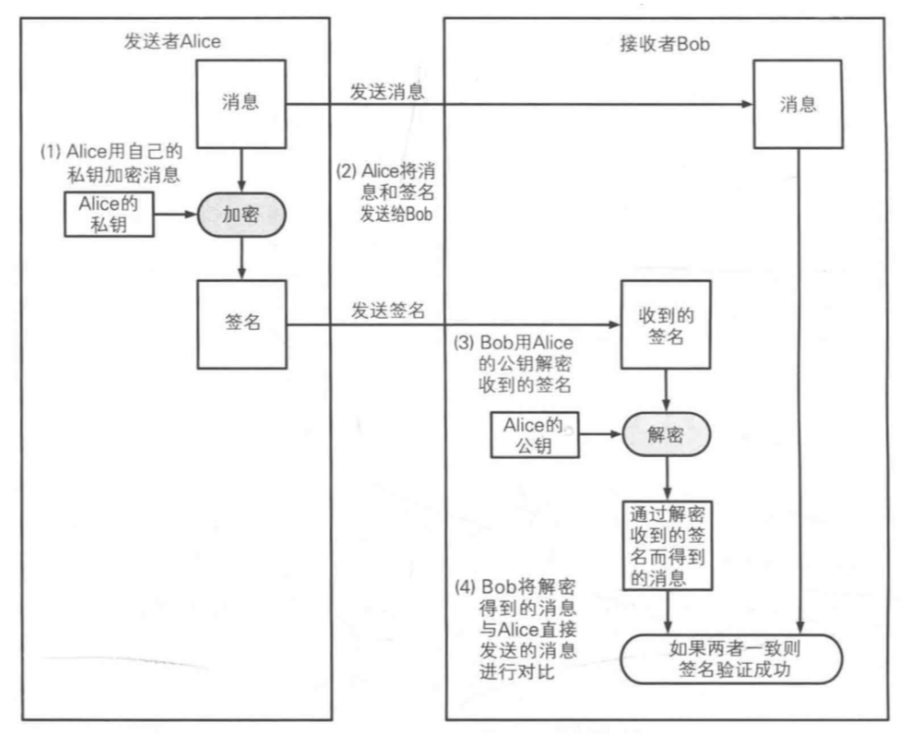#### 签名方案

1. 直接对消息签名
2. 对消息的散列值签名（计算更便捷）

#### 签名算法

1. RSA
2. DSA
3. ECC - 利用椭圆曲线密码来实现的数字签名算法

### 代码片段

#### RSA签名 & 验签

 ```func main() { //生成私钥 priv, _ := rsa.GenerateKey(rand.Reader, 1024) //通过私钥生成公钥 pub := &priv.PublicKey //通过hash散列对准备签名的名为做hash散列 plaitxt := []byte("hello world") //实现散列过程 h := md5.New() h.Write(plaitxt) hashed := h.Sum(nil) //通过pss函数，实现对明文hello world的签名 //pss函数可以加盐，能够使得签名过程更安全 opts := rsa.PSSOptions{rsa.PSSSaltLengthAuto, crypto.MD5} //实现签名 sig, _ := rsa.SignPSS(rand.Reader, priv, crypto.MD5, hashed, &opts) //sig就是RSA对“hello world”签名结果 fmt.Println(sig) //通过公钥实现验签 err := rsa.VerifyPSS(pub, crypto.MD5, hashed, sig, &opts) if err == nil { fmt.Println("验签成功") } }```

#### DSA签名 & 验签

DSA - 专业做数字签名的技术方案 - 不能用于加密和解密

 ```func main() { //设置私钥使用的参数 var param dsa.Parameters dsa.GenerateParameters(¶m, rand.Reader, dsa.L1024N160) //创建私钥 var pri dsa.PrivateKey pri.Parameters = param //生成私钥 dsa.GenerateKey(&pri, rand.Reader) //创建公钥 pub := pri.PublicKey message := []byte("hello world") //签名 r, s, _ := dsa.Sign(rand.Reader, &pri, message) //公钥验签 if dsa.Verify(&pub, message, r, s) { fmt.Println("验签成功") } }```

#### ECC（椭圆加密）签名 & 验签

ECC椭圆加密

• 由DSA改进而成
• 密钥可以比RSA更短，安全性可以比RSA更高
• 比特币就是用ecc作签名的
 ```func main() { message := []byte("hello world") //生成私钥 //elliptic.P256()设置生成私钥为256 privatekey, _ := ecdsa.GenerateKey(elliptic.P256(), rand.Reader) //创建公钥 publickey := privatekey.PublicKey //hash散列明文 digest := sha256.Sum256(message) //用私钥签名 r, s, _ := ecdsa.Sign(rand.Reader, privatekey, digest[:]) //设置私钥的参数类型 param := privatekey.Curve.Params() //获取私钥的长度(字节) curveOrderBytes := param.P.BitLen() / 8 //获得签名返回的字节 rByte, sByte := r.Bytes(), s.Bytes() //创建数组 signature := make([]byte, curveOrderBytes*2) copy(signature[:len(rByte)], rByte) copy(signature[len(sByte):], sByte) //现在signature中就存放了完整的签名的结果 //验签 digest = sha256.Sum256(message) //获得公钥的字节长度 curveOrderBytes = publickey.Curve.Params().P.BitLen() / 8 //创建大整数类型保存rbyte,sbyte r, s = new(big.Int), new(big.Int) r.SetBytes(signature[:curveOrderBytes]) s.SetBytes(signature[curveOrderBytes:]) //开始认证 e := ecdsa.Verify(&publickey, digest[:], r, s) if e == true { fmt.Println("验签成功") } }```